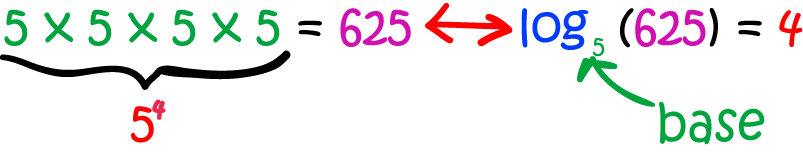# Definition of LogarithmThe logarithm $\log_b(a) =$ asks the question, "What power must I raise $b$ to so that I get $a$?"

For example, let's find $\log_5(625)$.

The question is, what power must we raise $5$ to so that we get $625$?

The answer is $625 = 5 \times 5 \times 5 \times 5 = 5^4$. So, the answer is $4$.

We say that the logarithm to the base $5$ of $625$ is $4$, and write $\log_5 (625) = 4$.

### Description

The aim of this dictionary is to provide definitions to common mathematical terms. Students learn a new math skill every week at school, sometimes just before they start a new skill, if they want to look at what a specific term means, this is where this dictionary will become handy and a go-to guide for a student

### Audience

Year 1 to Year 12 students

### Learning Objectives

Learn common math terms starting with letter L

Author: Subject Coach
You must be logged in as Student to ask a Question.